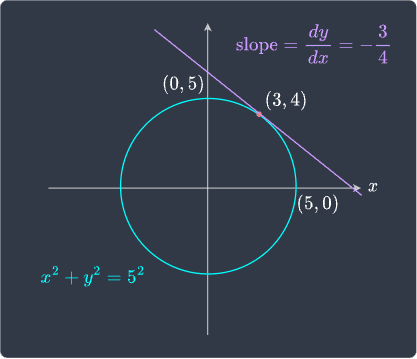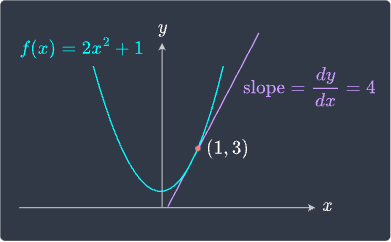search
Search
Unlock 100+ guides
search toc
close
Cancel
Post
account_circle
Profile
exit_to_app
Sign out
search
keyboard_voice
close
Searching Tips
Search for a recipe:
"Creating a table in MySQL"
Search for an API documentation: "@append"
Search for code: "!dataframe"
Apply a tag filter: "#python"
Useful Shortcuts
/ to open search panel
Esc to close search panel
to navigate between search results
d to clear all current filters
Enter to expand content previewDoc SearchCode Search BetaSORRY NOTHING FOUND!
mic
Start speaking...Voice search is only supported in Safari and Chrome.
Shrink
Navigate to
check_circle
Mark as learned
thumb_up
0
thumb_down
0
chat_bubble_outline
0
Comment
auto_stories Bi-column layout
settings

# Guide on Implicit Differentiation

schedule Aug 12, 2023
Last updated
local_offer
Calculus
Tags
mode_heat
Master the mathematics behind data science with 100+ top-tier guides
Start your free 7-days trial now!

# Motivating example

In Calculus, we are often given a function $f(x)$ and asked to take the derivative with respect to $x$. For instance, given $f(x)=x^2$, we can easily obtain the derivative $f'(x)=2x$. However, what if the given equation is not a function of such forms? For instance, consider the equation of the circle:

$$\begin{equation}\label{eq:Fslf5tE3Tagbblgeb9s} x^2+y^2=3^2 \end{equation}$$

This is clearly not of form $f(x)$, but we can rearrange the equation to make $y$ the subject:

$$\begin{equation}\label{eq:kRqwKaeXzuUpPstSdgE} y=f(x)=\pm\sqrt{3^2-x^2} \end{equation}$$

Even though \eqref{eq:kRqwKaeXzuUpPstSdgE} is of form $f(x)$, the problem here is that we have a $\pm$, which means we actually have two equations instead of just one. This is confusing and begs the question of which equation we should take the derivative of. This is where implicit differentiation comes into play. Instead of making $y$ the subject, we keep the equation \eqref{eq:Fslf5tE3Tagbblgeb9s} as is.

We know from \eqref{eq:kRqwKaeXzuUpPstSdgE} that $y$ is a function of $x$. Therefore, we can replace $y$ with $y(x)$ to emphasize this fact. Keep in mind that $y(x)$ does not mean $y$ multiplied by $x$, but rather $y$ as a function of $x$. Let's now rewrite \eqref{eq:Fslf5tE3Tagbblgeb9s} as follows:

$$\begin{equation}\label{eq:RiPNMSOl5TT3Q7hlEou} x^2+[y(x)]^2=3^2 \end{equation}$$

Taking the derivative of both sides gives:

$$\begin{equation}\label{eq:EcLD65bmk58YD91kH3g} \frac{d}{dx} \Big(x^2+[y(x)]^2\Big) =\frac{d}{dx}(3^2) \end{equation}$$

The right-hand side is easy - the derivative of a constant is $0$ like so:

$$\frac{d}{dx}(3^2)=0$$

Let's now tackle the left-hand side:

\begin{equation}\label{eq:M7T97SIY3o8KSyK7fLW} \begin{aligned}[b] \frac{d}{dx} \Big(x^2+[y(x)]^2\Big)&= \frac{d}{dx}x^2+\frac{d}{dx}[y(x)]^2\\ &= 2x+\frac{d}{dx}[y(x)]^2\\ \end{aligned} \end{equation}

We must use the chain rule when taking the derivative of $[y(x)]^2$ with respect to $x$ because $y(x)$ is a function of $x$ like so:

$$\begin{equation}\label{eq:o2VbzIulAuTBKLY79o4} \frac{d}{dx}[y(x)]^2= 2\cdot{y(x)}\cdot{y'(x)} \end{equation}$$

Substituting \eqref{eq:o2VbzIulAuTBKLY79o4} into \eqref{eq:M7T97SIY3o8KSyK7fLW} gives:

$$\begin{equation}\label{eq:AhETI3i0iVFLLxA00HN} \frac{d}{dx} \Big(x^2+[y(x)]^2\Big)= 2x+2\cdot{y(x)}\cdot{y'(x)} \end{equation}$$

We now substitute \eqref{eq:AhETI3i0iVFLLxA00HN} back into our equation \eqref{eq:EcLD65bmk58YD91kH3g}:

$$2x+2\cdot{y(x)}\cdot{y'(x)}=0$$

Now that we are done with taking the derivative, we can drop the $(x)$ part:

$$2x+2\cdot{y}\cdot{y'}=0$$

Finally, let's make $y'$ the subject:

$$y'=-\frac{x}{y}$$

Unlike the standard case, the derivative of $y$ with respect to $x$ is dependent on both on $x$ and $y$, rather than just $x$.

Example.

# Computing slope of tangent line of a circle using implicit differentiation

Consider a circle with radius $5$:

$$x^2+y^2=5^2$$

Compute the slope of the tangent line at $(3,4)$.

Solution. Let's first confirm that the point $(3,4)$ is indeed on the circle:

$$(3)^2+(4)^2=25=5^2$$

Great, so the point $(3,4)$ lies on the circle! From the previous section, we know that the derivative of $y$ with respect to $x$ for a circle is computed by:

$$\begin{equation}\label{eq:A1sPwr15aeUpKbhW5mL} y'=\frac{dy}{dx}=-\frac{x}{y} \end{equation}$$

Let's substitute $x=3$ and $y=4$ into \eqref{eq:A1sPwr15aeUpKbhW5mL} to get:

$$\begin{equation}\label{eq:uxJaG0WbWBkywFiQI3G} y'=\frac{dy}{dx}=-\frac{3}{4} \end{equation}$$

This is the slope of the tangent line at $(3,4)$!

Finally, let's illustrate our findings:Great, we're done!

Example.

# Performing implicit differentiation

Consider the following function:

$$-2x^2+y=1$$

Compute the slope of the tangent line at point $(1,3)$.

Solution. We will tackle this problem in two ways:

• standard way of differentiation

• implicit differentiation

Unlike the circle example, we can easily make $y$ the subject to get the $f(x)$ form:

$$y=f(x)=2x^2+1$$

Taking the derivative of $f$ with respect to $x$ gives:

$$y'=4x$$

Therefore, at point $(1,3)$, the slope of the tangent line is:

$$y'=4$$

Let's now tackle this problem using implicit differentiation. We take the derivative of both sides with respect to $x$ like so:

\begin{align*} \frac{d}{dx}\Big(-2x^2+y(x)\Big)&=\frac{d}{dx}(1)\\ -4x+y'&=0\\ y'&=4x\\ \end{align*}

Therefore, at point $(1,3)$, the slope of the tangent line is:

$$y'=4$$

This is the same as the slope computed using the standard way of differentiation!

Finally, let's visualize the tangent line:Great, we're done!

thumb_up
thumb_down
Comment
Citation
Ask a question or leave a feedback...
thumb_up
0
thumb_down
0
chat_bubble_outline
0
settings
Enjoy our search
Hit / to insta-search docs and recipes!